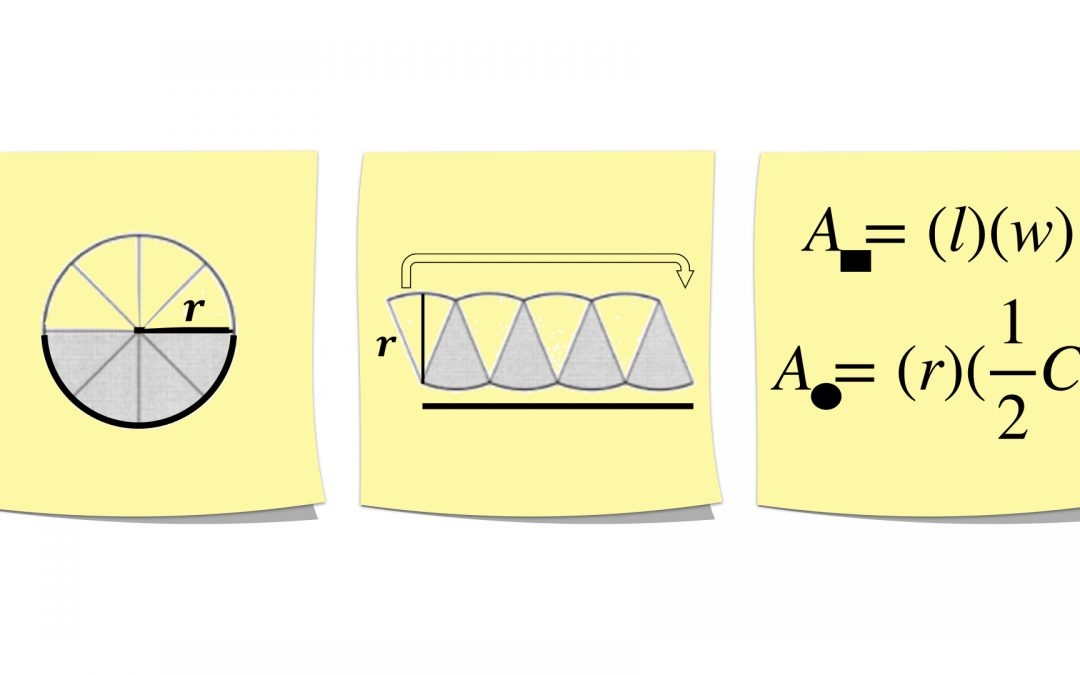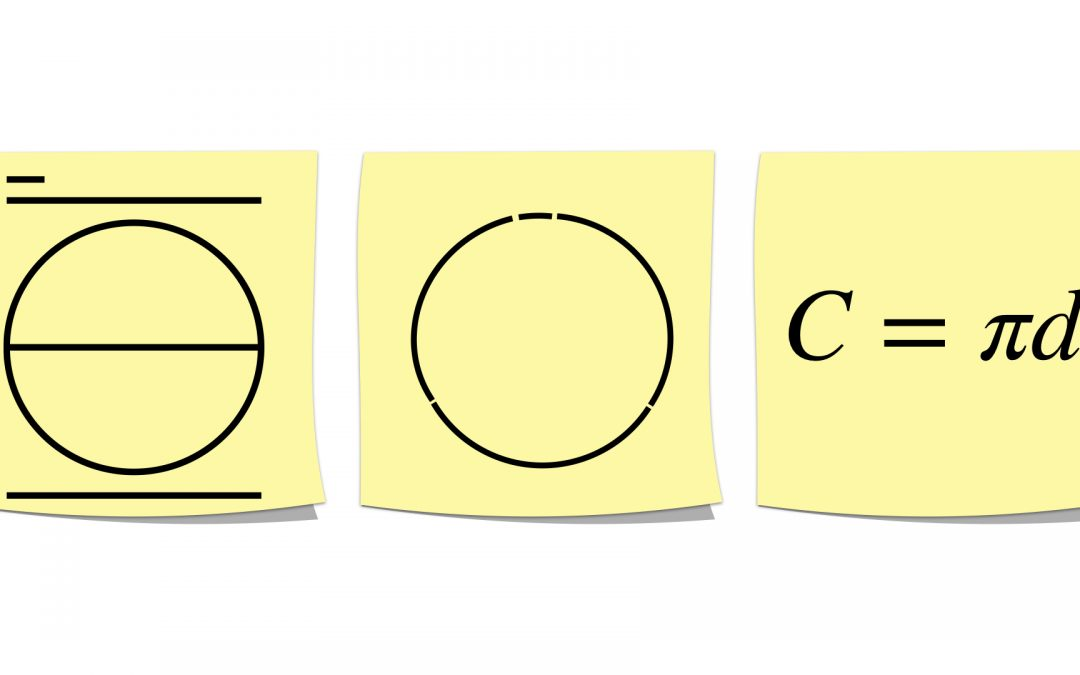Select Page## The Relationship between the Area of a Circle & its Circumference, Part Two: What does it Mean?

Common Core Standard: 7.G.B.4 In part one, I provided some Sticky Math Triads to put the process before students and teachers.  While I struggled there to provide visual representations, I believe I have accomplished the goal here as we examine the resulting...## The Relationship between the Area of a Circle and its Circumference, Part 1: Manipulating Formulas

Common Core Standard: 7.G.B.4 I have to confess that while I have done a lot of conceptual work with students around circumference and area of a circle (see some of it by checking out my blogposts on each subject), I have not done much to tackle the latter part of...## Area of a Circle Related to the Area of a Rectangle / Parallelogram

Common Core Standard: 7.G.B.4 If area is defined as the number of unit squares that cover the surface of a closed figure, then how does this apply to a circle? Can we remember the abstract formula for area of a circle better by understanding where it comes from...## Circumference of a Circle & the Meaning of Pi

Common Core Standard: 7.G.B.4 These Sticky Math activities can each stand alone; however, each of these blogposts suggests how you might put them together to form a lesson.  You can always adapt them to suit your needs. This Sticky Math Lesson helps build fluency from...

## Which One & Why?

Common Core Standards (different examples support different standards): 6.EE.A.2, 7.EE.A.1, 8.F.A.2, 8.F.A.3, HSA.SSE.A.1.A, HSF.IF.B.4 Besides using Sticky Math to compare two different representations or match representations, you could provide two nonequivalent...